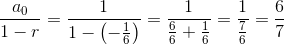# High School Math : Sequences and Series

## Example Questions

1 3 Next →

### Example Question #2 : Finding Partial Sums In A Series

Find the sum of all even integers fromto.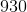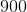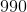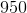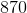Explanation:

The formula for the sum of an arithmetic series is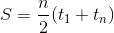,

whereis the number of terms in the series,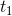is the first term, and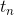is the last term.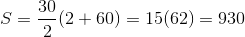### Example Question #3 : Finding Partial Sums In A Series

Find the sum of the even integers from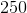to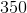.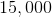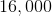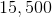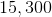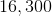Explanation:

The sum of even integers represents an arithmetic series.

The formula for the partial sum of an arithmetic series is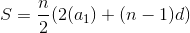,

where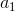is the first value in the series,is the number of terms, andis the difference between sequential terms.

Plugging in our values, we get: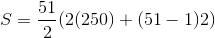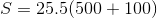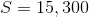### Example Question #1 : Sums Of Infinite Series

Find the value for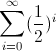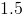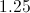Explanation:

To best understand, let's write out the series. So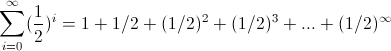We can see this is an infinite geometric series with each successive term being multiplied by.

A definition you may wish to remember is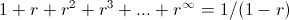where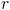stands for the common ratio between the numbers, which in this case isor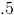. So we get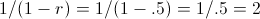### Example Question #2 : Sums Of Infinite Series

Evaluate: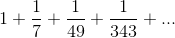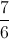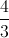The series does not converge.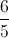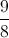Explanation:

This is a geometric series whose first term is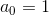and whose common ratio is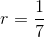. The sum of this series is: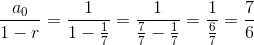### Example Question #3 : Sums Of Infinite Series

Evaluate: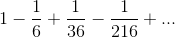The series does not converge.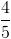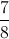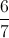This is a geometric series whose first term isand whose common ratio is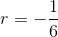. The sum of this series is: